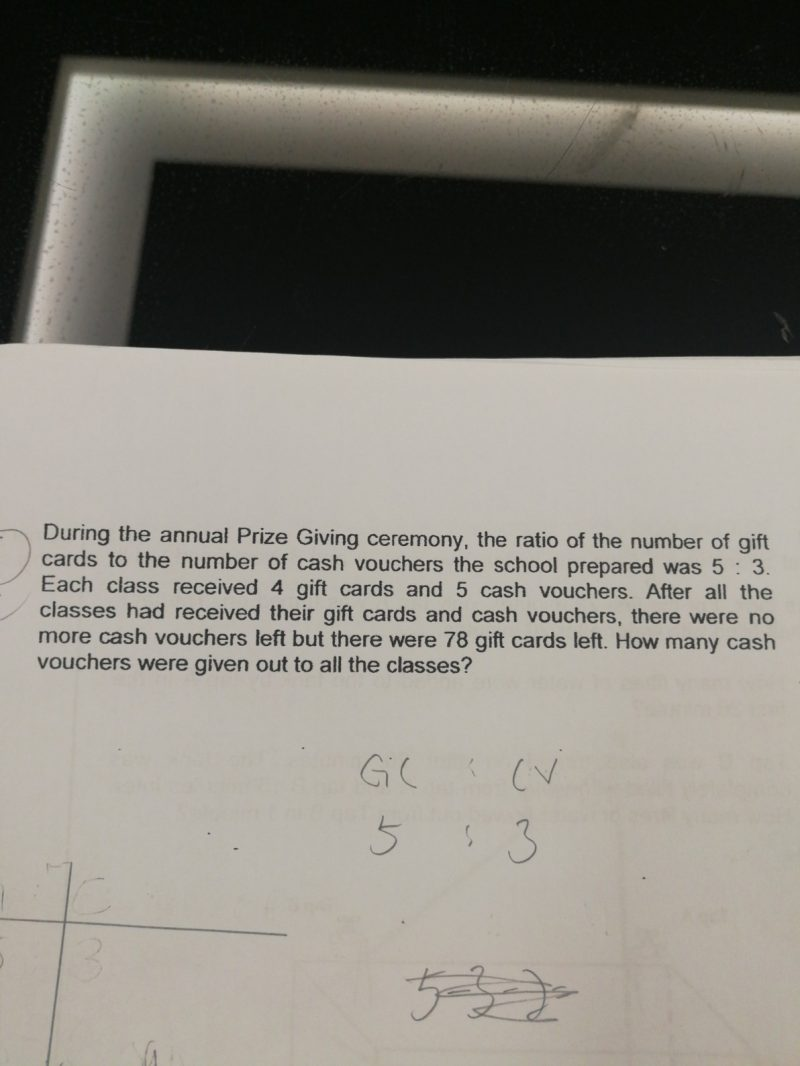# QuestionCan someone help my Gal with this question ? Thanks

1 Answer

# Answer

School > GC :CV = 5 : 3 = 25 : 15

Class > GC : CV = 4 : 5 = 12 : 15

25 units – 12 units = 13 units

13 units = 78

1 unit = 6

15 units = 90

90 cash vouchers were given out to all the classes.

0 Replies 0 Likes